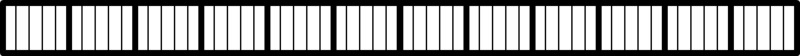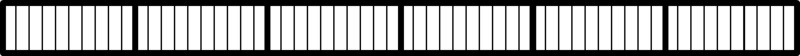Analyzing Word Problems Involving Multiplication

Alignments to Content Standards: 3.OA.A.3

Many problems can be solved in different ways. Decide if the following word problems can be solved using multiplication. Explain your thinking. Then solve each problem.

1. Liam is cooking potatoes. The recipe says you need 5 minutes for every pound of potatoes you are cooking. How many minutes will it take for Liam to cook 12 pounds of potatoes?
2. Mel is designing cards. She has 4 different colors of paper and 7 different pictures she can glue on the paper. How many different card designs can she make using one color of paper and one picture?
3. Nina can practice a song 6 times in an hour. If she wants to practice the song 30 times before the recital, how many hours does she need to practice?
4. Owen is building a rectangular tile patio that is 4 tiles wide and 6 tiles long. How many tiles does he need?

IM Commentary

The purpose of this task is for students to analyze different contexts in which multiplication is appropriate. Research indicates that students often do not make sense of word problems. Instead, to come up with an answer, they just apply the most recent algorithm taught, chose operations based on the types of numbers involved, or apply other approaches that do not require sense making. In this task, the students are asked to analyze the problems and explain their thinking before they find an answer. In the process, they are thinking more carefully about different contexts that can be represented by multiplication.

Note that none of the contexts involve multiplicative comparison, which is intentionally left until 4th grade (see 4.OA.A.1).

Solutions

Solution: Analysis

1. While you can solve this by repeated addition (5+5+5+….), this problem can also be solved by multiplication since adding 5 together 12 times is the same as $12\times5$.
2. This can be solved by multiplication, because for each of the four colors, there are seven different pictures from which to choose, so 7 pictures on the first color + seven pictures on the second color + 7 pictures on the third color + 7 pictures on the fourth color gives four groups of seven or $4\times 7$.
3. This is a division problem since the number of hours needed can be determined by counting the number of groups of six needed to make 30, or $$30 \div 6 = ?$$ On the other hand, we can think about this in terms of multiplication by asking what times six equals 30, which we can write symbolically as $$? \times 6 = 30$$
4. It is a good idea to draw a picture:We can count the number of tiles one by one, or we can see that there are four rows, each with six tiles, so we can think of it as a multiplication problem $4 \times 6$.

Solution: Solving explicitly

1. $12\times5$ is 12 groups of 5, which we can see in the picture below:12 groups of 5 is the same as 6 groups of 10:which is 60. So Liam needs 60 minutes to cook the potatoes.

2. An array makes it easier to see:There are 28 different possible cards.

3. If we multiply the number of hours she practices by 6, we know the answer must be 30: $$?\times 6 = 30$$ Since 5 times 6 is 30, she has to play for 5 hours if she wants to practice the song 30 times.
4. Looking at the picture above, we can see that Owen needs 24 tiles.